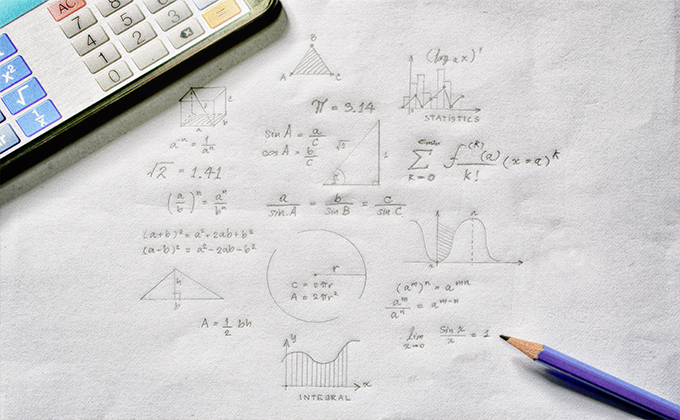×The Free 30-Day CAT RC Course

"It is designed to help you excel in the upcoming CAT 2023 exam"

-By Lavleen Kaur Kapoor. Over 1,73,000 Subscribers

No thanks >

OPEN

## IBSAT, CAT, XAT, NMAT, GMAT

OPEN#### Get Personalized MBA Mentorship

Connect with my team on WhatsApp for unbiased MBA College Selection advice.

# Tricky Questions on Banker's DiscountCAT Registration Ends: 13th September 2023### Theoretical Concept:

Suppose a merchant Ram buys goods worth. say Rs.1,00,000 from another merchant Shyam at a credit of say 10 months. Then, Shyam prepares a bill, called the bill of exchange. Ram signs this bill and allows Shyam to withdraw the amount from his bank account after exactly 10 months.

The date exactly after 10 months is called nominally due date. Three days {known as grace days) are added to it get a date, known as legally due date.

Suppose Shyam wants to have the money before the legally due date. Then he can have the money from the banker or a broker. Who deducts S.I. {Simple Interest) on the face value (i.e., Rs.1,00,000in this case) for the period from the date on which the bill was discounted (i.e., paid by the banker) and the legally due date. This amount is known as Banker's Discount {B.D.).

Thus, B.D.is the S.I. on the face value for the period from the date on which the bill was discounted and the legally due date.

Banker's Gain {B.G.) = {B.0.) - (T.D.) for the unexpired time.

Note: When the date of the bill is not given, grace days are not to be added.

### Important Formulas:

i)  B.D.= S.I. on bill for unexpired time.

ii) B.G.= {B.D.).(T.D.) = S.I. on T.D.= (T.D.)*P.V.

iii) T.D.=  P.W. x B.G.

iv) B.D.= [(Amount x Rate x Time)/100]

v) T.D.= ({Amount x Rate x Time)/ 100 + (Rate x Time)] vi) Amount = ((B.D.x T.D.)/ B.D.*T.D]

vii) T.D.= ((B.G. x 100)/ (Rate x Time)]

### Solved Illustrations:

Question 1)

The banker's discount on a bill due 4 months hence at 15%is Rs. 420.The true discount is:

 A. Rs.400 B. Rs.360 c. Rs.480 D. Rs.320

Solution:

T.D.= B.D. x 100/ 100 + (R x T)

= Rs. [420 x 100/100 + (15*1/3))

= Rs.420 x 100/ 105 = Rs.400

Question 2)

The banker's discount on Rs.1600 at 15% per annum is the same as a true discount on Rs. 1680 for the same time and at the same rate. The time is:

 A. 3 months B. 4 months c. 6 months D. 8 months

Solution:

S.I. on Rs.1600 = T.D. on Rs.1680.

Rs.1600 is the P.W. of Rs.1680, i.e., Rs.80 is on Rs.1600 at 15%. Time = {100 x 80/ 1600 x 15} Years = 1/3 years = 4 months

Read More : CAT Quantitative Aptitude (QA)

Question3)

The banker's discount on a sum of money for 11/2 years (one and half years) is Rs.558 and the true discount on the same sum for 2 years is Rs. 600.The rate percent is:

 A. 10% B. 13% c. 12% D. 15%

Solution:

B.D. for 3/2 years = Rs.558.

B.D. for 2 years = Rs.558.2/3.2 = Rs.744

T.D. for 2 years = Rs.600.

Therefore, sum = B.D.*T.D./B.D.- T.D.=Rs. [744 *600/144) = Rs.3100

Thus, Rs.744 is 5.1. on Rs.3100 for 2 years.

Therefore, Rate = [(100 *744)/ (3100 x 2)) % = 12%

Question 4)

The banker's gain on a certain sum due 11/2 year hence is 3/2!5 of the banker's discount. The rate percent is:

 A. 5 1⁄2 % B. 9 1⁄11 % c. 8 1⁄8 % D. 6 1⁄6 %

Solution:

let, B.D = Re.1.

Then. B.G.= Re.3/25

T.D.= {B.D.- B.G.) = Re. {1- 3/25) = Re.22/25 Sum = [1x {22/25)/ 1-{22/25)) = Re.22/3

S.I. on Rs.22/3 for 11/2 years is Re.1

Rate = [100 x 1/ {22/3*3/2)) % = 100/11= 9 1/11%

Read More : QA Study material - Important Concepts and Formulas on Clock Problems

Question 5)

The banker's discount on a certain sum due 2 years hence is 11/10 of the true discount. The rate percent is:

 A. 11% B 10% c. 5% D. 5.5%

Solution:

let T.O. be Re.1.

Then,8.0. = Rs.11/10 = Rs.1/10

Sum = Rs. ((1.10 x 1)/ {1.10 - 1)) = 110/10 = Rs.11

S.I. on Rs.11 for 2 years is Rs.1.10

Rate = ((100"1.10}/ (11*2)) % = 5%

Attempt CAT Mock Test Now

Read Complete Module on Quantitative Ability

Stay informed, Stay ahead & Stay inspired with MBA Rendezvous